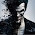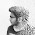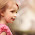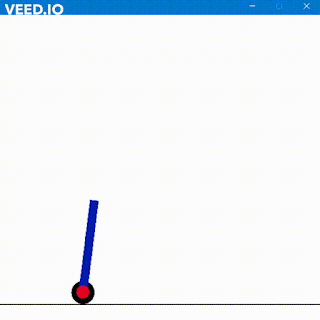### Verilog Code for Hamming Code Detector

Today I am going to discuss Hamming Code, its parity working and its code detection. Hamming code detection is basically used when there is a single bit error. It is a quite fast and efficient method to determine the error bit and replace it with the opposite bit.

Let us take data as – 01001101 and with parity, it will be xx0x100x1101. How are the parity bit set here comes in mind first.

The parity bit is always set at positions which are a power of 2 like 1, 2, 4, 8, 16 etc. So

As you can see the parity bits are set as X at positions of power of 2. Follow same process for any data.
Now, what will be at these positions?

Note down position 1 as P1 position 2 as P2 position 4 as P4 and position 8 as P8. Now for P1 start from position 1. Take the first bit and ignore next and again take another and ignore next. So P1 will be x01010. Similarly for P2 starting bit will be at position 2 so take two bits at once then ignore the next two bit and again take two and ignore the next two. So P2 will be x00010

Similarly, for P4 starting bit position will be 4 so take 4 bits at once then ignore next 4 then take another 4 and ignore next 4. So P4 will be x1001. Similarly, for P8 we will have P8 as x1101 since we only have 5 bits left from position 8.

Now P1 has even number of 1s hence its parity is 0 thus P1 = 0. Similarly, P2 has an odd number of 1s so odd parity hence P2 = 1.

Similarly P4 has even number of 1s so P4 = 0

And P8 = 1

Now the data with parity become by replacing P1-8 as 010010011101.

This is our Hamming Code.

Now let there be an error and the data with parity become this 010010011111. The red bit was 0 but by noise, it becomes 1. Now follow the same procedure to find P1-8

Now P1 has position 1, P2 has position 2, P4 has position 4, and P8 has 8. So add the positions whose match is “NO”.  1 + 2 + 8 = 11 Thus 11th bit has the error and we have to replace that bit. Now go and check the position of the colored red bit. Voila its 11

Here is the code for Hamming code

Here is the Output

So Long

1.2.Thanks So much...neatly explained.

1.3.Will you please give me the verilog code for error position correction in hamming code.

1.2.3.Thank you for responding to me.
Suppose the actual encoded data is 10101010000,while transmission of data due to noise there is an error position on '5',the received data is 10100010000.
Kindly give me a verilog code for the above and correcting that position.

4.Have a look at the above post. It is the same what you require.

5.Also In the output you can clearly see the position of error of bit, noise_data and actual data too

4.This comment has been removed by the author.

5.can you provideme verilog code for multiple bit errors?

1.Give him some time
He will surely provide
It appears he is a busy man

6.This comment has been removed by the author.

7.the program is always detecting 11th bit even though we change the data in testbench program

### Self Balancing Robot using Machine Learning

Hola Amigos, I have always loved inverted pendulums. They are very fascinating to me and I play with them a lot. In real life, I have m...